• Category

# Top 10 Data Visualization Techniques

• Riya Kumari
• Mar 03, 2021
• Updated on: Jun 15, 2021The tendency of human eyes is to get attracted more towards visuals rather than written content. You may also have faced this situation where you felt easier to understand through visuals like charts, graphs, etc.

Thus, data visualization comes in handy as it organizes the raw data into an easier visual format. It can help by providing data in the very efficient possible way.

## Data Visualization

Data visualization gives a fast and productive way to convey the message in a widespread way by using visual information. It is used in almost all industries to improve sales with existing customers and also target new markets and demographics for possible customers.

Uses of data visualization:

• The major use is the preprocessing part of the data mining procedure.

• It is an influential way to analyze data with presentable outcomes.

• It plays a role in mixing sectors as part of the data reduction process.

• It helps the process of data cleaning by locating inaccurate and missing values.

Simply, we can say that data visualization gives a clear idea about what raw information means through visuals in a way that is universal, and effective. There are different types of techniques you can use to visualize data that will be discussed in the blog.

(Also read: Visualizing Geospatial data with Kepler.gl)

## Data Visualization Techniques

1. ### Box plots

A box plot or box and whisker plot give a visual outline of information through its quartiles.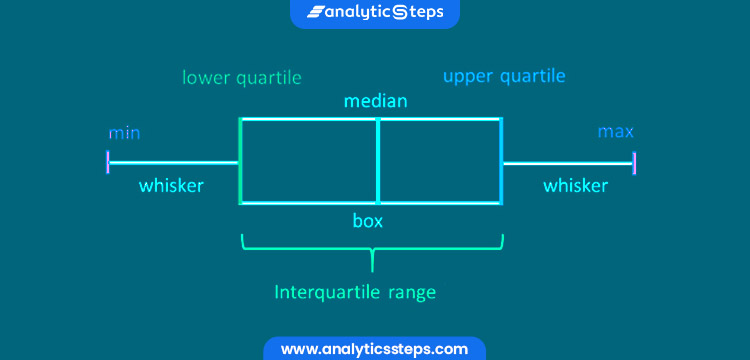Box Plot Visualization

• At first, a box is drawn from the primary quartile to the third of the data set. A line inside the box addresses the median.
• "Whiskers," or lines, are then drawn stretching out from the box to the base (lower extreme) and greatest (upper extreme).
• Outliers are addressed by individual focuses that are in-line with the whiskers.
• This kind of outline is useful in rapidly distinguishing whether the information is balanced or slanted, just as giving a visual rundown of the data set that can be effectively deciphered.

In simple language, we can understand that box plots indicate the five-number summary of a set of data which comprises the minimum score, lower quartile, median, upper quartile, and maximum score.

(Recommended blog: 4 Types of Data Visualization Using R Programming )

1. ### Histograms

A histogram is a graphical presentation of information using bars of various heights and in a histogram, each bar groups numbers into ranges.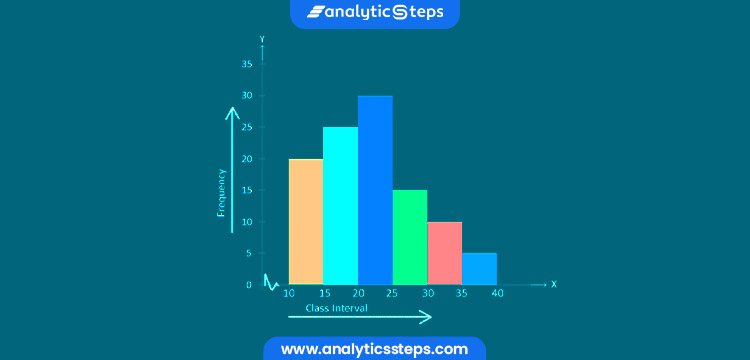Sample Histogram Graph

• Taller bars indicate that more data falls in that range.
• A histogram shows the shape and spread of continual sample data.
• It is a plot that allows you to find, and show, the basic frequency distribution (shape) of a set of continuous data.
• This permits the assessment of the data for its essential distribution, skewness, outliers, and so on.
• It is an exact portrayal of the distribution of mathematical data and it relates just a single variable.
• Incorporates bin or bucket- the range of values that partition the whole range of values into a progression of intervals and afterward check the number of values that fall into every interval.
• Bins are sequential, non-covering intervals of variables. As the adjacent bins leave no gaps, the rectangles shapes of the histogram contact each other to demonstrate that the first value is continuous.

(Most related: Statistical data distribution models)

1. ### Heat maps

A heatmap has a very different concept of representing the data. It is a graphical portrayal of data that uses different colors to address different values. This difference in color representation makes it easy for the viewers to understand the trend more quickly.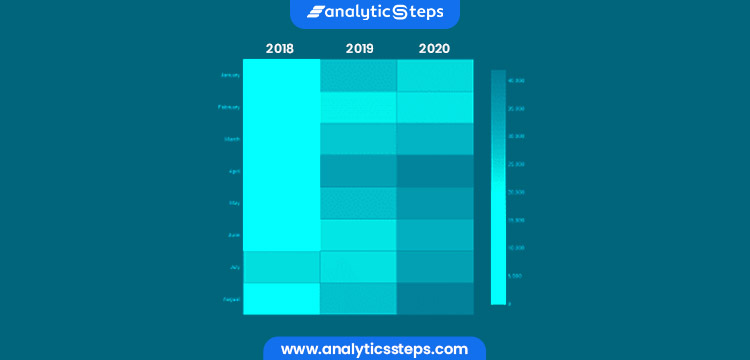Heat Maps

It is beneficial for two major purposes:

1. For visualizing correlation tables
2. For visualizing missing values in the data

In both cases, the information is communicated in a two-dimensional table.

For instance, if you need to dissect which time of day a store makes the most deals, in that case, you can use a heat map that indicates the day of the week on the vertical axis and time of day on the horizontal axis.

After that, by shading in the matrix with colors that relate to the number of deals at each time of the day, you can specify the trends in the data that enable you to decide the specific times your store experiences the most deals.

1. ### Charts

There are several types of charts:

• #### Bar Charts

It is one of the simple techniques of data visualization. These types of charts are used to compare the quantities of different categories.

Hence, values of a category are addressed with the aid of bars and they can be designed with vertical or flat bars, with the length or height of each bar addressing the value.

If you want to examine data over time or the data is assembled in multiple sectors like different industries, variety of food, etc, a Bar Graph is the best option with some characteristics or some sorts of thorough ideas.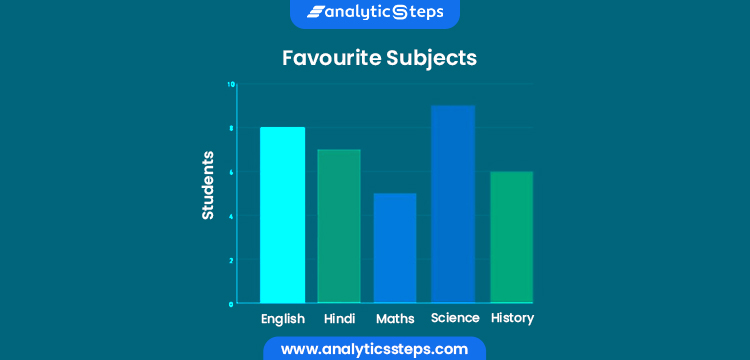Bar Charts

• #### Line Charts

It is used to plot the relationship of dependence of one variable on another like if you want to show data over very long periods or continuously changing data, the line graph could be a solid option to consider.

To plot the connection between the two variables, we can basically call the plot function. The line chart is most often used to indicate trends and evaluate how the data has changed over time.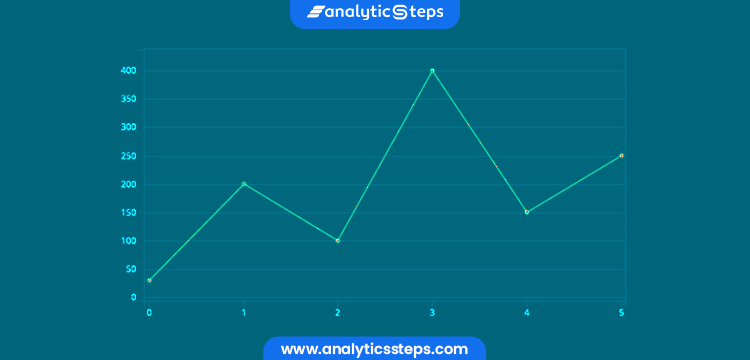Line Charts

• #### Pie Charts

Pie Chart is one of the very basic and well-known techniques of data visualization. It is very simple and easy to understand. It is a circular statistical graph that supposes pieces to clarify numerical ratios. Thus, here the arc size of each piece is equal to the amount it indicates.

For example, a company witnessed a growth of 150% in which they found out 60% of growth was due to marketing, 40% was due to sales, 30% was due to product and 20% was due to technology adoption.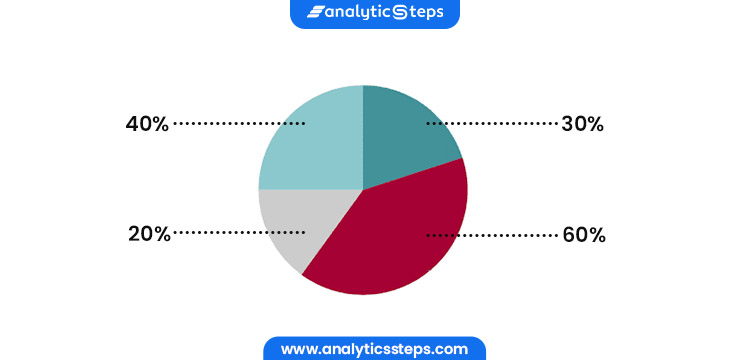Pie Charts

• #### Scatter Charts

It is a two-dimensional plot denoting the joint variation of two data elements such that

1. Each marker like a dot, plus signs indicates an observation.
2. The marker position implies the value for each observation.

Simply, it is a type of mathematical illustration that shows the value for generally two variables for a set of data by using Cartesian coordinates.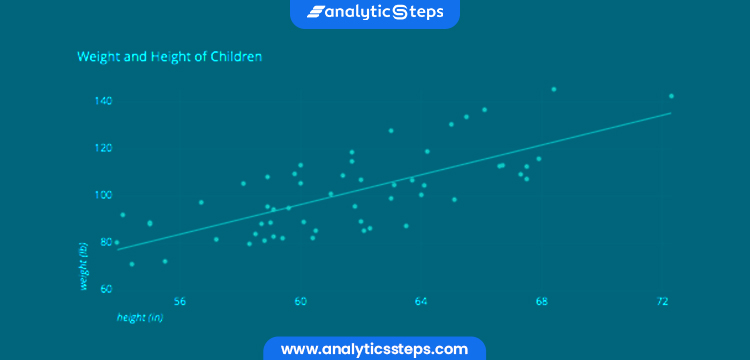Scatter Charts

• #### Bubble Charts

Bubble charts are a variation of scatter charts in which the data points are replaced with bubbles. Also, an extra proportion of data is portrayed in the size of the bubbles. You can use this chart for analyzing patterns or correlations.

Each dot in a bubble chart adapts with a single data point. The variables’ values for each point are implied by horizontal position, vertical position, and dot size.Sample Bubble Charts

1. ### Treemaps

This method indicates hierarchical data in a nested format, (understand how hierarchical clustering works)Sample Treemaps

• In Treemap, the size of the rectangles used for each category is proportional to its percentage of the whole.
• A leaf hub rectangle has a zone corresponding to the predetermined element of the data.
• Depending upon the decision, the leaf hub is colored, sized, or both as per picked credits.
• They utilize space, hence show a great many things on the screen all the while.

1. ### Word Cloud and Network diagram for Unstructured Data

The assortment of big data brings difficulties because semistructured and unstructured information requires new visualization techniques.

A word cloud visual addresses the frequency of a word inside a collection of text with its general size in the cloud. This technique is used on unstructured data as an approach to show high-or low-recurrence words.Word Cloud

Another visualization technique that can be used for semistructured or unstructured information is the network diagram. As discussing about network diagram, learn about network grpah and network topology)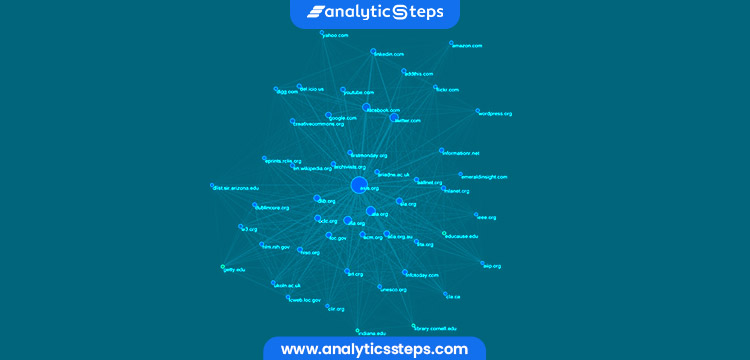Network Diagram

• The network diagram addresses connections as nodes and ties.
• They are used in numerous applications, for instance, for the investigation of social networks or mapping item deals across geographic territories.

(Most related: What is Knowledge graph?)

1. ### Wedge stack graphs

Wedge stack graphs are one of the techniques of data visualization that shows hierarchical data in a radial system.Wedge stack graphs

• These graphs can be used to illustrate multilevel frequency data.
• If you request a stacked graph with wedges then the graph type shifts to Walls that are stacked.
• The object size and number of side indicators do not affect the Wedges graph type.

1. ### Correlation matrices

A correlation matrix enables quickly recognizable proof of connections between variables by joining enormous information and quick reaction times.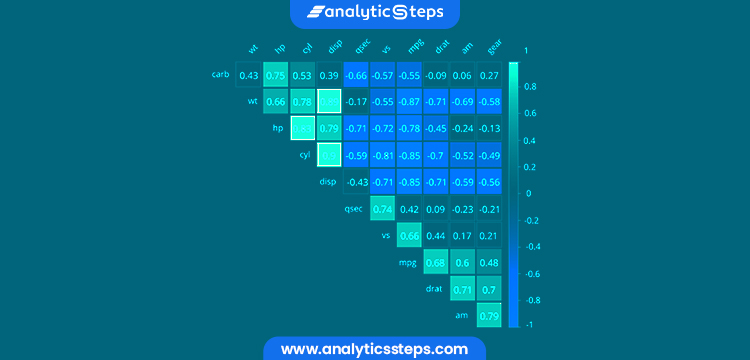Correlation matrices

• Essentially, a correlation matrix is a table showing correlation coefficients between variables.
• Each cell in the table illustrates the connection between two variables.
• The correlation matrix is used as an approach to sum up the data, as a contribution to a further developed investigation, and as a demonstrative for cutting-edge analyses.
• Generally, we use correlation matrices as inputs for exploratory factor analysis, confirmatory factor analysis, structural equation models, and linear regression when excluding missing values pairwise.

1. ### Streamgraphs

A streamgraph is a variety of stacked area charts. Rather than plotting values against a customary y-axis, the streamgraph balances the baseline of each "stack" to make it even around the x-axis.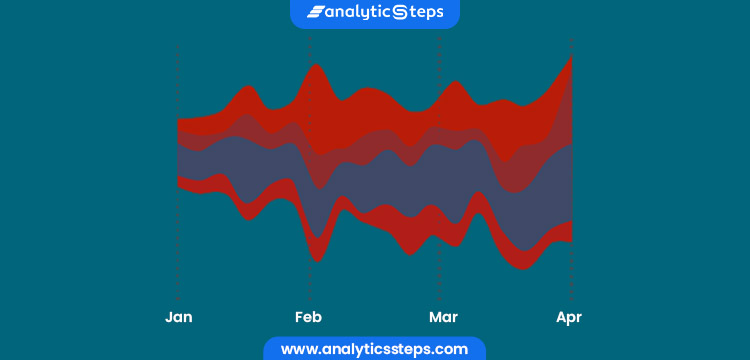Streamgraphs

• Stream Graphs are ideal for showing high-volume datasets, to find patterns and trends over the long haul across a wide scope of classifications.
• For instance, seasonal peaks and troughs in the stream shape can propose an intermittent example.
• A Stream Graph could likewise be used to picture the instability for a huge gathering of resources throughout a specific timeframe.

1. ### Dendrograms

Dendrograms show the hierarchical connection between the objects. The major use of a dendrogram is to figure out the best path to allocate objects to clusters.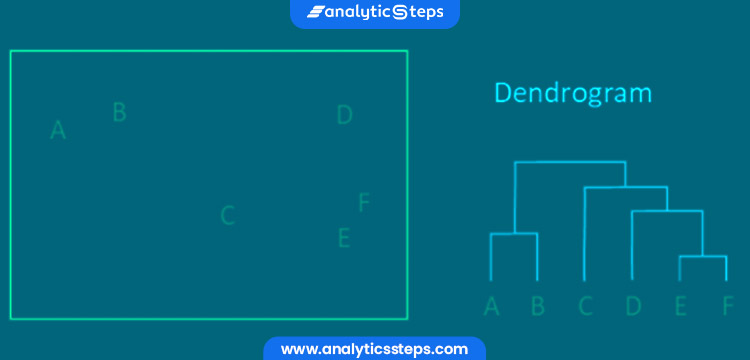Sample picture of Dendrograms

• The key to inferring a dendrogram is to point on the height at which any two objects are joined together.
• In the above example, we can see that E and F are most similar, as the height of the link that joins them together is the smallest, and the next two most similar objects are A and B.

Two types of dendrogram exist which results in 2 types of the dataset:

1. A hierarchical dataset gives the links between nodes explicitly.
2. The outcome of a clustering algorithm can be visualized as a dendrogram.

( Also read: Power BI and Tableau: Data Visualization Tools)

•#### innoart.seo

Jun 30, 2022

Some good techniques of data visualization and the info is helpful. Thank you for sharing.

•